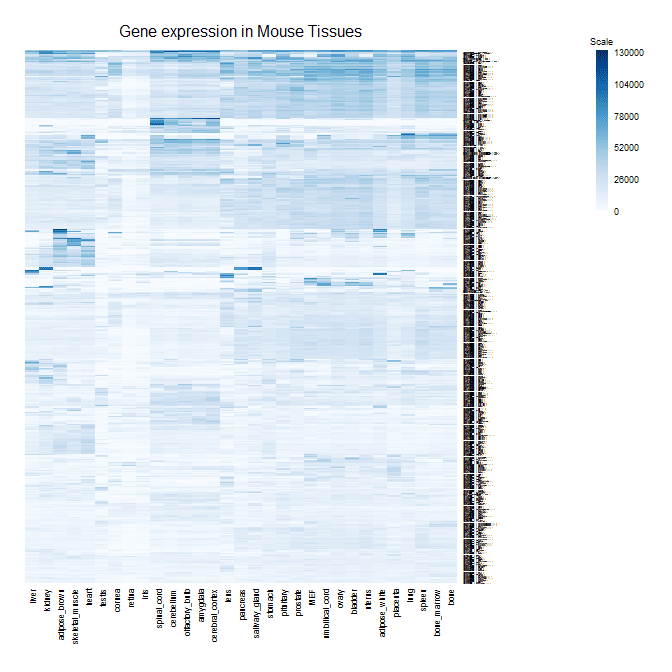Key With Heatmap
2
4
Entering edit mode
11.8 years ago
Diana ▴ 900

Hi, I am making heatmaps using R. The heatmap function in R does not support a key with the heatmap. Does anyone know how to give a key with the heatmap based on colours? Many thanks

heatmap r • 18k views
9
Entering edit mode
11.8 years ago

My general answer would be to use image(). I never really liked the way heatmap.2 looked.

Here's my image plot function with a corresponding color scale.

mcm_image<-function(data,colors,title="",zlim=c(floor(min(data,na.rm=T)),ceiling(max(data,na.rm=T))),addgrid=F,cexCol=.8,cexRow=.1,...)
{
library(RColorBrewer)
par(mar=c(7,2,4,7))
image(t(data[nrow(data):1,]),axes=F,col=colors,zlim=zlim)

{
abline(v=seq(-1*seq(0,1,length.out=ncol(data))/2,1+seq(0,1,length.out=ncol(data))/2,length.out=ncol(data)+1),lwd=.1,col='white')
abline(h=seq(-1*seq(0,1,length.out=nrow(data))/2,1+seq(0,1,length.out=nrow(data))/2,length.out=nrow(data)+1),lwd=.1,col='white')
}

axis(1, at=seq(0,1,length.out=ncol(data)),labels=colnames(data),tick=F,las=2,cex.axis=cexCol,line=-.5)
axis(4, at=seq(0,1,length.out=nrow(data)), labels=rev(rownames(data)), tick=F,las=2,cex.axis=cexRow,line=-.5)
mtext(title,side=3,outer=F,cex=1,line=1)

labels <- seq(zlim,zlim,length.out=6)
par(mar=c(1,4,4,5))
axis(4,at=seq(0,1,length.out=6),tick=F,labels=round(labels,digits=0),cex.axis=.9,las=2,line=-.5)
mtext("Scale",side=3,outer=F,cex=.6,line=.4)
}
colorBar(data,colors,zlim);

}

library(RColorBrewer)
h<-heatmap(as.matrix(data))
nf<-layout(matrix(data=c(1,1,1,2,0,0),nrow=3,ncol=2),widths=c(13,3),TRUE)
layout.show(nf)
mcm_image(data=as.matrix(data)[h$rowInd,h$colInd],colors=colorRampPalette(brewer.pal(9,"Blues")((100),title="Gene Expression in Mouse Tissues",zlim=c(0,130000),addgrid=F)0
Entering edit mode

That looks good!! thanks a lot Madelaine

0
Entering edit mode

hello,

suppose that i have already downloaded GSE63706 and normalized that and i have a normalized text file now. and i have also a list of probsets (a text file of my interest probsets) in this array...i want to have a heat map showing the expression pattern of my interest probsets in this array, for example in this array i have 4 varieties and different tissues (rind and flesh) and phases (0,10,20,30,40 and 50 days after harvesting). heat maps showing the expression pattern of my probsets in varieties, tissues and phases i mean

4
Entering edit mode
11.8 years ago
Philippe ★ 1.9k

Hi,

have you tried the heatmap.2 function? It's part of the gplots package and provide a enhanced version of the basic heatmap function. By default, the key is present.

You can see its documentation page there and you can find many additional resources on Google (or even Biostar) if you are still stuck.